# How to Generate all Prime Numbers between two given Numbers in Excel?

Excel is a famous spreadsheet-based tool, used to manipulate and process the available data. For this provided scenario, user need to evaluate the prime number between the two provided numbers. This tutorial includes two examples, first example is based on the user define formula. For this example, user need to define formula header. While for the second example user defines a module by using a VBA code. This VBA code can be called by using a explicit method.

## Let’s show you Some Examples

### Example 1

Step 1

Consider the below given sample workbook. This excels sheet contains two number first one contains data for start number, while the second row contains last number as a prime number range: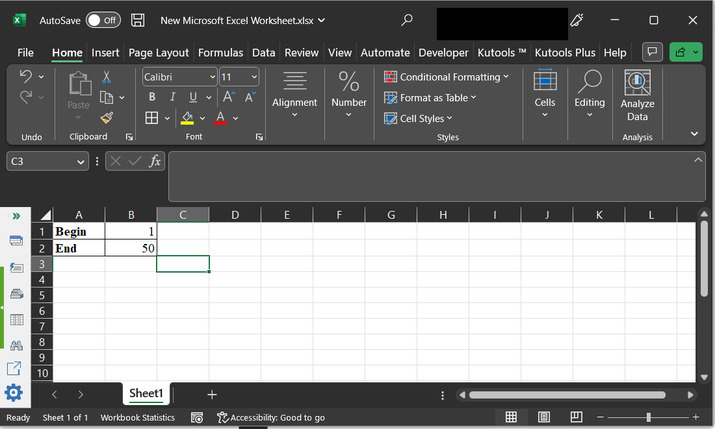Step 2

After that go to the Formula tab, and under the “Defined Names” section, select the “Name Manager”. Consider the below depicted image: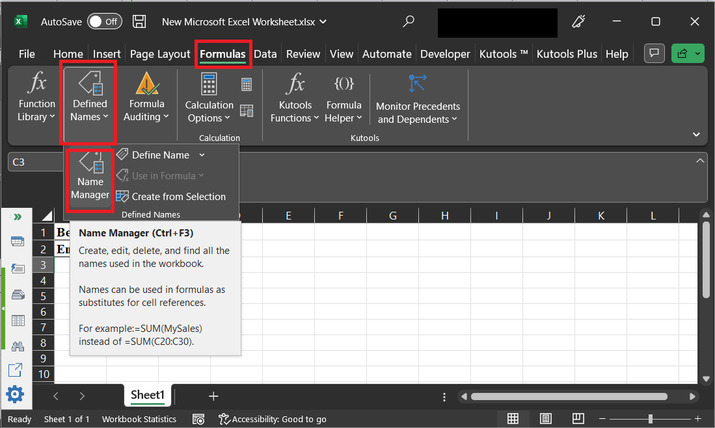Step 3

This will open the below given dialog box: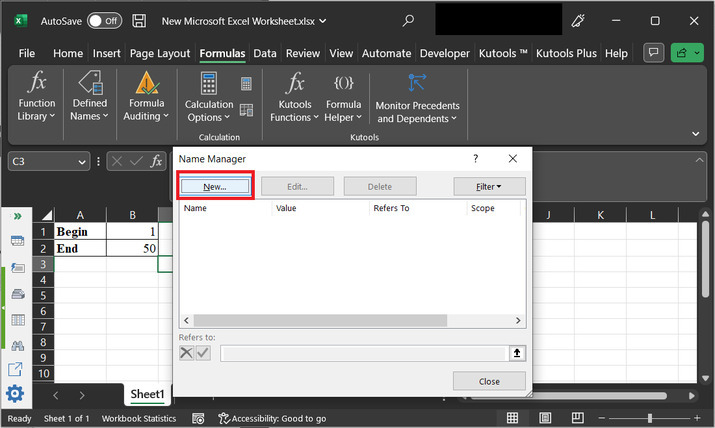Step 4

Click on “New” button. This will open the below provided dialog box: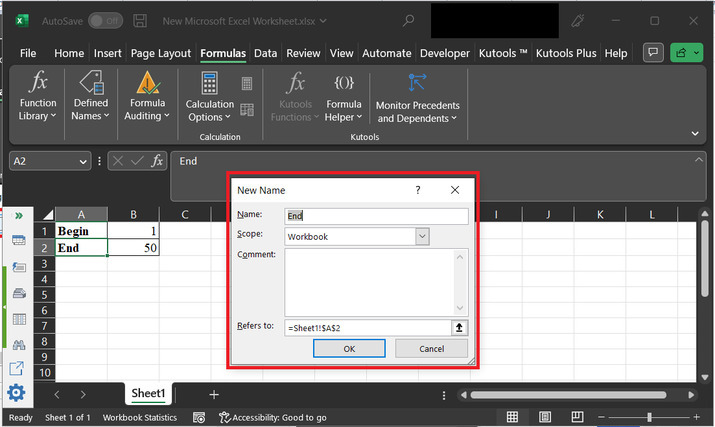Step 5

Change name to “prime_range”, and in the “refers to” column simply add, the formula “=ROW(INDIRECT(Sheet1!$B$1&":"&Sheet1!$B$2))”.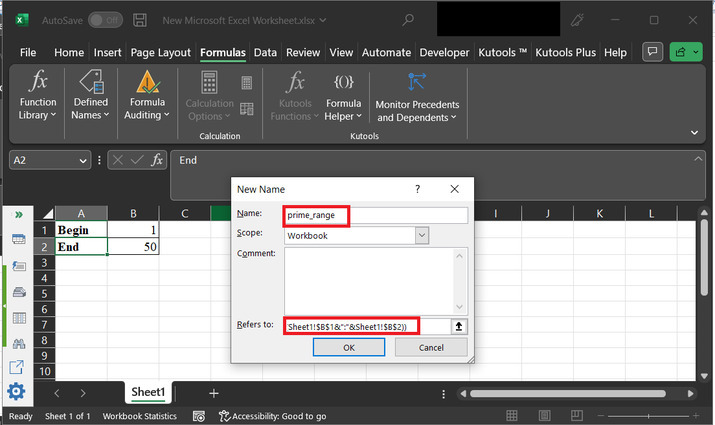Step 6

Click on “OK”. This will open the below given dialog box: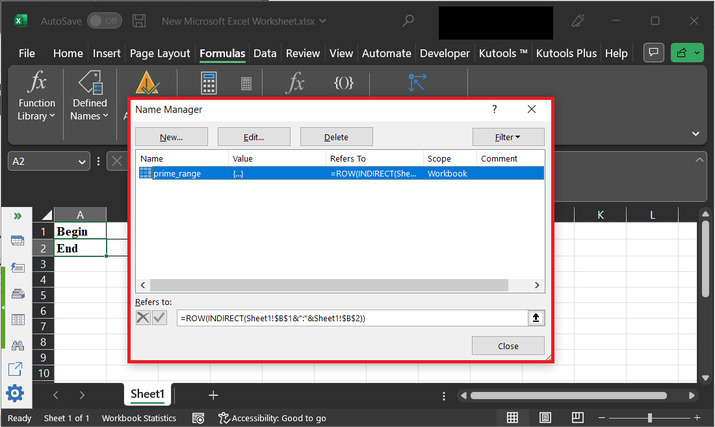Step 7

Again, click on “New" option.Step 8

Go to the name option and type “prime”. After that click on “refers to”, and paste the provided formula:

=SMALL(IF(MMULT(--(IF(prime_range >TRANSPOSE(prime_range -Sheet1!$B$1+2),MOD(prime_range,( prime_range >TRANSPOSE(prime_range -Sheet1!$B$1+2))*TRANSPOSE(prime_range -Sheet1!$B$1+2)))=0), prime_range -Sheet1!$B$1+2)=0, prime_range),ROW(INDIRECT("1:"&Sheet1!$B$2)))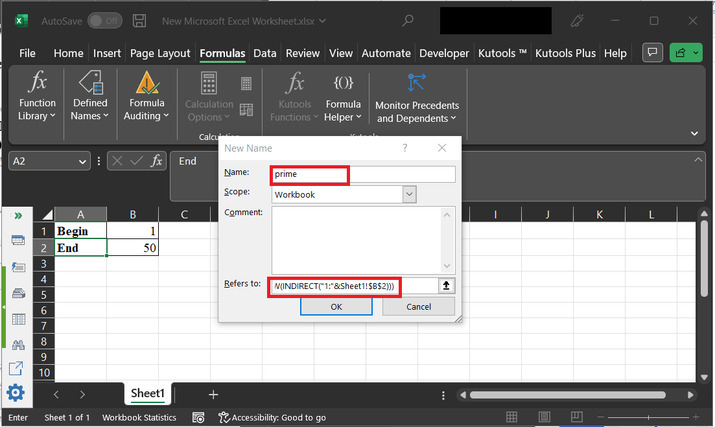Step 9

Click on “OK”. This will open the below depicted dialog box: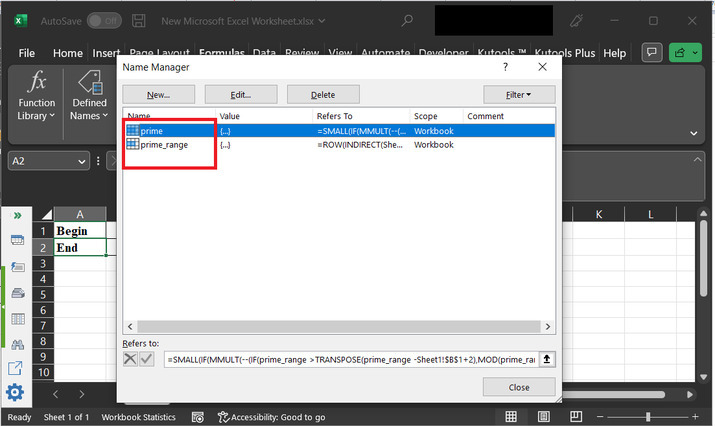Step 10

A Click on “Close”.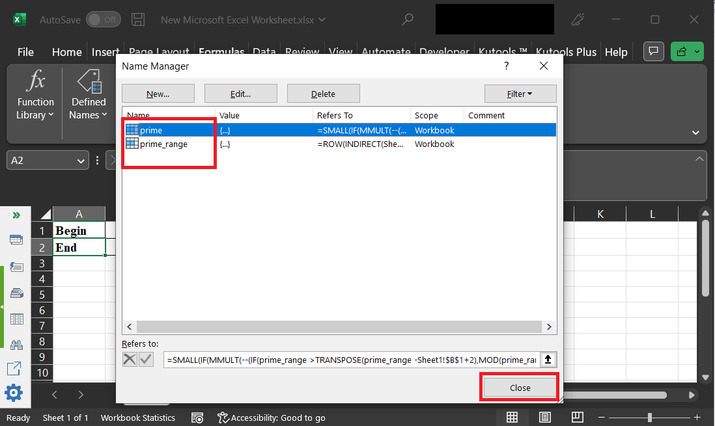Step 11

Go to the cell “D2” cell, and type formula “=IFERROR(prime,"")” .Press “Enter” key and the data will appear as given below: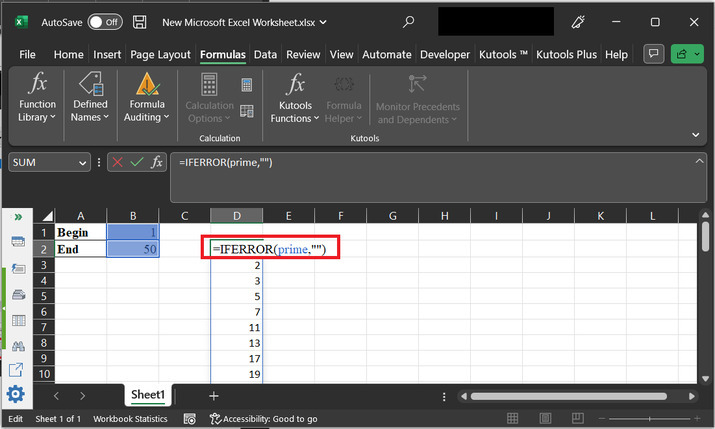Finally generated results are given below: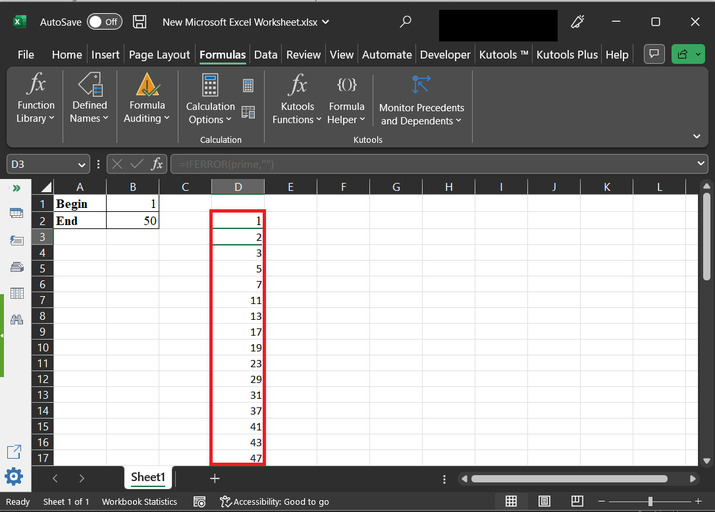### Example 2

Step 1

In this example, let’s use VBA to achieve same task.

Go to the “developer” section, and under the “code” section, click on “Visual basic”.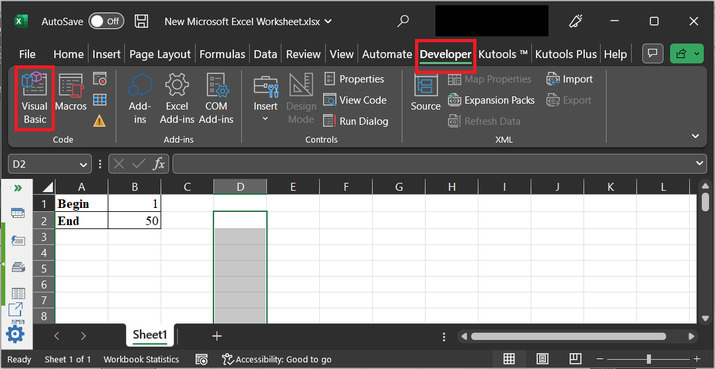Step 2

This will open below depicted dialog box: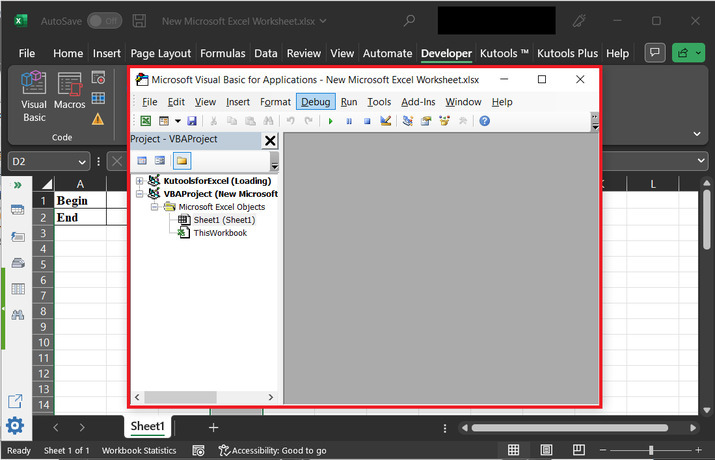Step 3

After that select “Insert”, and click on “module”.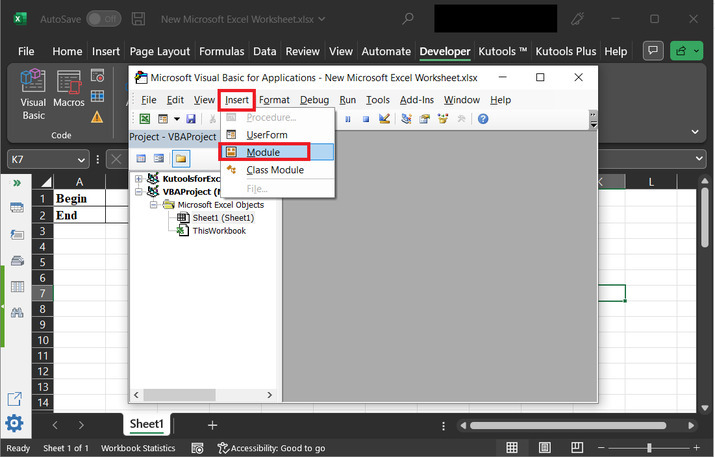Step 4

This will open the below given code area: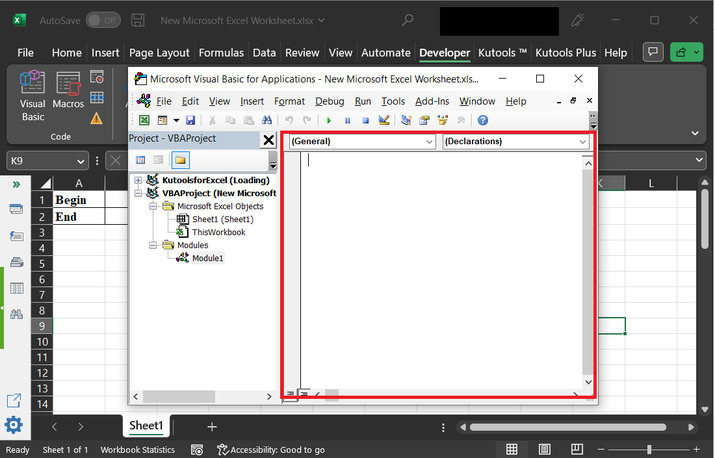### Copy below given code'define module Function Prime(Strt, last As Long) 'declaring required variables Dim value As String 'nested for loop expression For val_a = Strt To last ' inner for loop expression For val_b = 2 To val_a - 1 ' if block If val_a Mod val_b = 0 Then GoTo jump_label: ' next statement Next val_b ' processing step value = value & val_a & "," jump_label: ' next instruction Next val_a ' assign value to prime Prime = value ' end of module definition End Function 

Step 5

Snapshot for code area: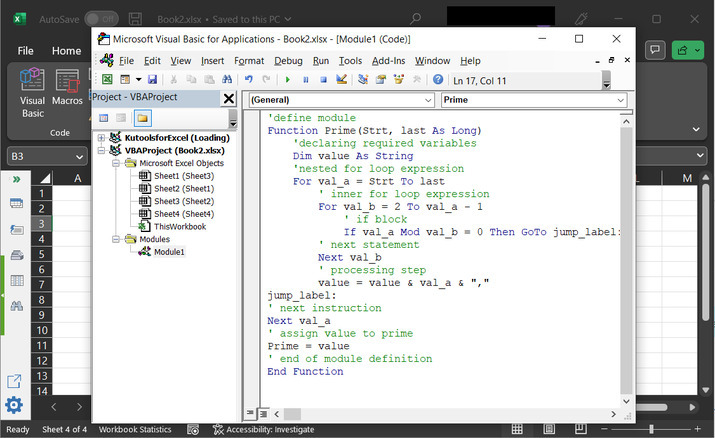Step 6

After that go back to the worksheet, and type “=prime(1,100)” on sheet. This will print prime number on sheet.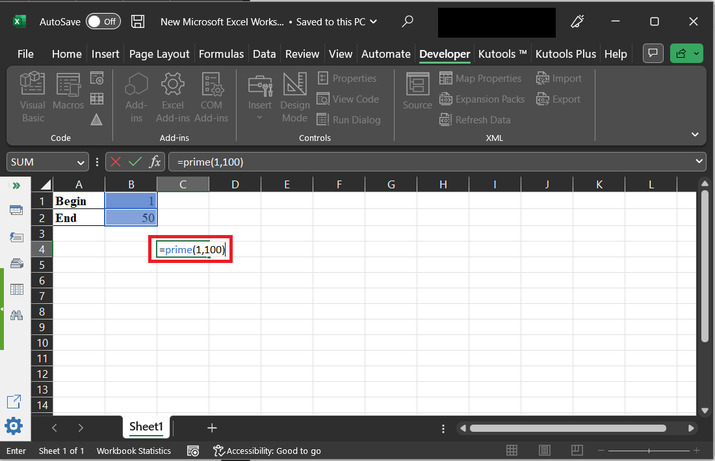Step 7

This will display the below given prime numbers on console: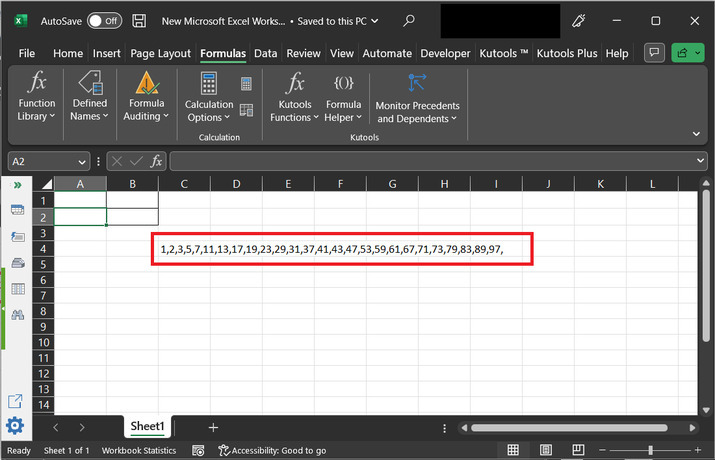## Conclusion

In this tutorial two simple examples, are used to explain “how to generate all the prime numbers between the two numbers in excel”. After performing all the above provided steps user will be able to understand the proper steps required to use while developing formula for prime number and while developing code for prime number.

Updated on: 22-Aug-2023

60 Views